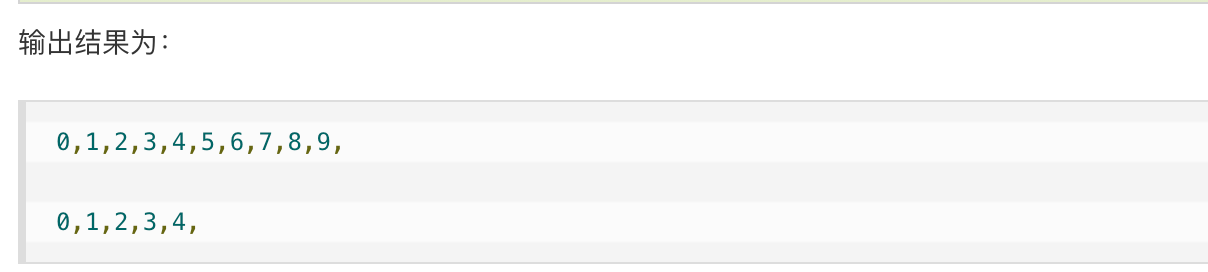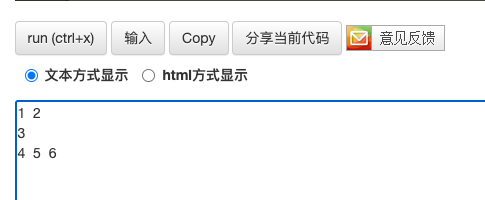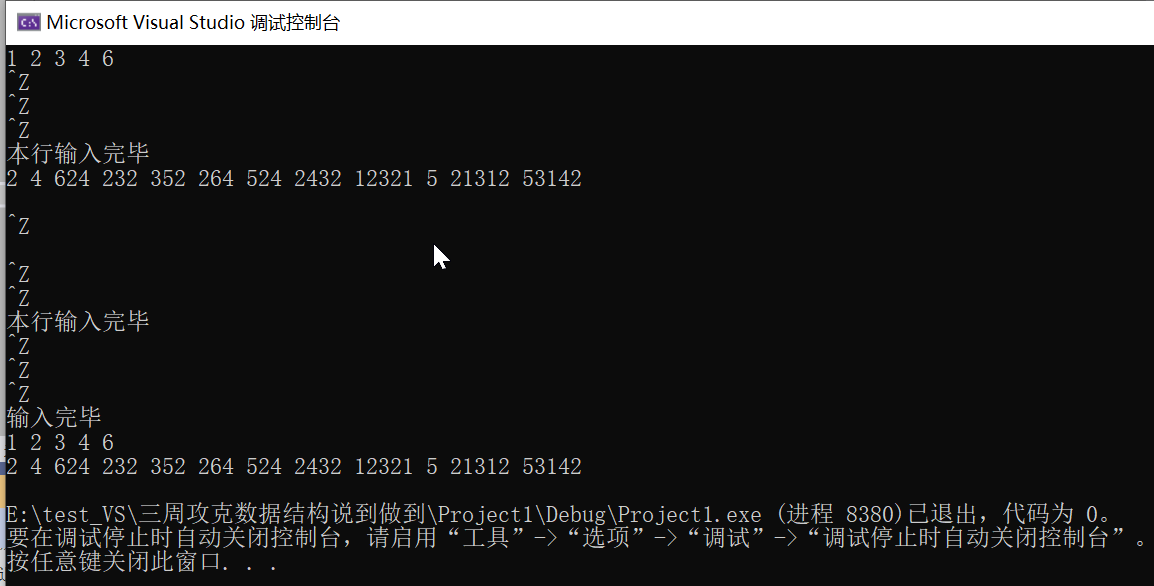• C++ 获取std::vector长度 大小

千次阅读 2021-10-08 21:46:44
vector> #include <iostream> using namespace std; int main() { vector<int>obj;//创建一个向量存储容器 int for(int i=0;i<10;i++) // push_back(elem)在数组最后添加数据 { obj.push.....

obj.size()

#include <string.h>
#include <vector>
#include <iostream>
using namespace std;

int main()
{
vector<int>obj;//创建一个向量存储容器 int
for(int i=0;i<10;i++) // push_back(elem)在数组最后添加数据
{
obj.push_back(i);
cout<<obj[i]<<",";
}

for(int i=0;i<5;i++)//去掉数组最后一个数据
{
obj.pop_back();
}

cout<<"\n"<<endl;

for(int i=0;i<obj.size();i++)//size()容器中实际数据个数
{
cout<<obj[i]<<",";
}

return 0;
}展开全文c++
• vector> using namespace std; //目标就是完成二维vector的所有操作 int main(void) { vector<vector<int>> vec2; //二维数组的 vector 定义 // 构造一维的vector vector<int> vec1;...
#include<iostream>
#include<vector>

using namespace std;

//目标就是完成二维vector的所有操作
int main(void)
{

vector<vector<int>> vec2; //二维数组的 vector 定义

// 构造一维的vector
vector<int> vec1;

vec1.push_back(1);
vec1.push_back(2);
// 将vec1插入到vec2中
vec2.push_back(vec1);

vec1.clear();
vec1.push_back(3);
vec2.push_back(vec1);

vec1.clear();
vec1.push_back(4);
vec1.push_back(5);
vec1.push_back(6);
vec2.push_back(vec1);

//打印“二维数组”，本处演示先按列打印
for(int i = 0; i < vec2.size(); ++i){
for(int j = 0 ; j< vec2[i].size(); ++j){
cout << vec2[i][j] << "  ";
}
cout << endl ;
}
}展开全文c++
• 我们可以参考之前写的一篇文章: 任意一维数组的输入输出 ...vector> #include<cstdio> using namespace std; //vector<vector<int>> matrixReshape(vector<vector<int&

我们可以参考之前写的一篇文章:

任意一维数组的输入输出

那么,我们可以借鉴之前的思路,使用两个scanf("%d",&temp)来嵌套输入,这样一来思路就很明确了.

#include<iostream>
#include<vector>
#include<cstdio>
using namespace std;

//vector<vector<int>> matrixReshape(vector<vector<int>>& mat, int r, int c) {
//
//}

int main()
{
int temp = 0;
vector<int> one;
vector<vector<int> > two;
while (scanf_s("%d", &temp) != EOF) {
one.push_back(temp);
while (scanf_s("%d", &temp) != EOF)
{
one.push_back(temp);
}
cout << "本行输入完毕"<<endl;
two.push_back(one);
one.clear();
}
cout << "输入完毕" << endl;
for (int i = 0; i < two.size(); ++i)
{
for (int j = 0; j < two[i].size(); ++j)
{
cout << two[i][j] << " ";
}
cout << endl;
}
return 0;
}

执行结果如下:补充:^z在键盘上的输入对应着ctrl+Z,在VS2019上对应着三次ctl+Z=EOF

展开全文c++ 开发语言 后端
• size()方法用于返回此Vector的大小(即元素存在的数量)。size()方法是一个非静态方法，只能通过类对象访问，如果尝试使用类名访问该方法，则会收到错误消息。返回size时，size()方法不会引发异常。语法：...

向量类size()方法size()方法在java.util包中可用。

size()方法用于返回此Vector的大小(即元素存在的数量)。

size()方法是一个非静态方法，只能通过类对象访问，如果尝试使用类名访问该方法，则会收到错误消息。

返回size时，size()方法不会引发异常。

语法：public int size();

参数：它不接受任何参数。

返回值：

方法的返回类型为int，它返回此向量的大小。

示例//Java程序演示示例

//size()Vector的int方法的说明

import java.util.*;

public class SizeOfVector {

public static void main(String[] args) {

//实例化Vector对象

//初始容量为“ 10”"10"

Vector  v = new Vector  (10);

//此v中的元素

//通过使用size()methos是返回

//大小，即元素数量存在

//显示向量大小

System.out.println("v.size(): " + v.size());

}

}

输出结果v.size(): 3

展开全文• 我把一个Message[]存在Vector和ArrayList都试了，都只能保存16个Mesage，但是我一共有27个，每个Message的大小不定，有3M的，也是20多K的，请问把怎么才能都保存在Vector或ArrayList，哪个效率更高一些呢？...
• vector段错误

2021-10-30 17:17:07
数组 在c语言中，我们说数组是随机存取的，存取的方式就是下标索引法。即假如我们要存或取一个数组的第n个元素，方法是一致的，都是“数组名[n-1]”。...当我们没有提前声明vector的容量时，计算机会给..c++ 开发语言 后端
• 您可以使用linspace和round生成索引：vector = [0 25 50 75 100 125 150]; % // datan = 4; % // desired number of equally spaced entriesind = round(linspace(1,length(vector),n)); %// get rounded equall...
• vector<vector<int>> points = { {1, 3},{2, 6},{3,7} }; for (vector<vector<int>>::iterator it = points.begin(); it != points.end(); it++) { for (vector<int>::iterator it...
• 一维数组 二维数组 vector<int> res(10); vector<vector<int>> res(10,vector<int>(10,INT32_MAX))c++
• vector> using namespace std; int main() { vector<int> v1; vector<int> v2; v1.push_back(1); v1.push_back(2); v1.push_back(3); v2 = v1; for(auto it=v2.begin(); it!=v2.end(); it++) ...
• 描述：长度为n的数组arr，进行以下操作：选择最小的arr进行+1操作，输出一个正整数表示执行完k次操作之后最小的最大值 输入：n k(数组长度 操作次数 3 3) 1 2 3(数组值) 输出：3 说明：第一次操作选择arr 令...c++ c语言 算法
• 很多初学者分不清楚 vector 容器的容量(capacity)和大小(size)之间的区别，甚至有人认为它们表达的是一个意思。本节将对 vector 容量和大小各自的含义做一个详细的介绍。vector 容器的容量(用 capacity 表示)，指的...
• 我想求arr 的实际长度是多少，没找到方法，自己写了一个方法，不知道是否可行。 int arr; arr = 1; arr = 2; arr = 3; // 模拟数据，int数组arr 实际长度 为 3 int len = end(arr)-begin(arr)...c++
• 在内存中，vector将元素连续存储，而vector的size也会随着我们添加的元素的增多而变大。不同于原生的数组需要我们在声明时就得指定数组的大小，这无疑给我们的开发工作带来了很多便利，那么，vector这个类究竟在底层...
• VHDL是一种硬件描述语言(HDL) . 您的std_logic_vector由...所以使用你需要的'MAX'长度的std_logic_vector，然后只读取你需要的位：constant zeros : std_logic_vector(MAX-1 downto 0) := (others => '0');sign...
• Lambda 表达式排序 // 从小到大排序 sort(records.begin(), records.end(), [](string a, string b){ return a.length() < b.length(); }); 自定义静态函数 static bool cmp(string a, string b){ ...c++ 排序算法 leetcode
• 但是，我接触到string、vector这些类类型时，根据它们的特性：它们能动态扩充，就是你来多少内容，我都能存的下！ 然后，我就想：那它们的变量大小是不是也扩充了，那是不是也导致所处的结构体的变量的大小也改变...
• Vector实现了AbstractList抽象类和List接口,和ArrayList一样是基于Array存储的Vector 是线程安全的,在大多数方法上存在synchronized关键字//Vector存放的元素,初始化默认长度为10protected Object[] elementData;...
• EigenvalueVector

2021-02-06 18:16:01
Chap6.dspChap6.dswChap6.ncbChap6.optEigenvalueVectorHessenbergQR.cppEigenvalueVectorRealSymmetryJacobi.cppEigenvalueVectorRealSymmetryJacobiB.cppEigenvalueVectorRealTriangleQR.cppHessenbergTransform.c...eigen向量计算
• java.util.Vector.setSize()方法可用于在Java中设置Vector的大小。如果设置的新大小大于Vector的旧大小，则将空值添加到创建的位置。如果设置的新大小小于Vector的旧大小，则将丢弃位置大于新大小的元素。演示此过程...
• C++ vector容器详解目录vector容器的基本概念1.vector的构造函数2.vector的赋值操作3.vector的容量与大小4...1.数组是静态的，长度不可改变，而vector可以动态扩展，增加长度 2.数组内数据通常存储在栈上，而vector中数容器 c++
• 因为对于vector和string，其部分的实现渗透到了接口中。 连续容器元素是连续存储的、容器大小是可变的 size是指容器已经保存元素的数目 capacity是不分配新的内存空间的前提下，容器最多可以保存多少元素
• vector本来就是可以用来代替一维数组的，vector提供了operator[]函数，可以像数组一样的操作，而且还有边界检查，动态改变大小。这里只介绍用它来代替二维的数组，二维以上的可以依此类推。1、定义二维vectorvector ...
• ##一、 获取两点距离的两种方式 直接获取距离 get ...1.获取对象位置，再获取vector length，然后将获取的对象位置的xyz 分别除以向量长度 组合成归一化后的的xyz 2.获取对象位置，然后直接使用normalize节点对
• C# Vector3

千次阅读 2020-12-18 10:52:32
Vector3 三维向量 表示3D的向量和点 Vector3.Angle 角度 由from和to两向量返回一个角度。形象的说，from和to的连线和它们一个指定轴向的夹角 public Transform target; void Update() { Vector3 targetDir = ...c#
• vector

2021-01-02 09:35:17
6、定义vector，如果要用到下标初始化，就需要提前定义vector的大小。vector与数组十分相似，唯一不同的是，向量在需要扩展大小的时候，会自动处理它自己的存储需求，比如使用push_back() C++关于vector的官方文档 ...c++ 开发语言 后端
• vector是C++标准模版库(STL,Standard Template Library)中的部分内容。之所以认为是一个容器，是因为它能够像容器一样存放各种类型的对象，简单的说：vector是一个能够存放任意类型的动态数组，能够增加和压缩数据。...
• 在 c++ 中，vector 是一个十分有用的容器。它能够像容器一样存放各种类型的对象，简单地说，vector是一个能够存放任意类型的动态数组，能够增加和压缩数据。C++ 中数组很坑，有没有类似 Python 中 list 的数据类型呢...
• 标准库Vector类型使用需要的头文件：#includeVector：Vector 是一个类模板。不是一种数据类型。 Vector是一种数据类型。一、 定义和初始化Vectorv1; //默认构造函数v1为空Vectorv2(v1);//v2是v1的一个副本Vectorv3(n...
• 一：直接上码演示 #include<... //vector的初始化 vector<int> v1(date1,date2),date1表示vector的大小 // date2表示vector的初始值是多少 vector<int> v1(10,10); int sum1c++...